# Chapter 18 Areas of Circle, Sector & Segment RS Aggarwal Solutions Exercise 18A Class 10 Maths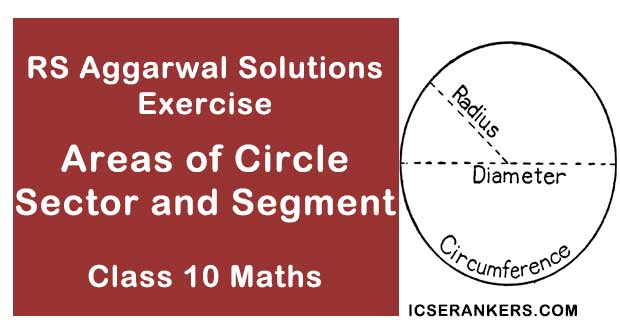Chapter Name RS Aggarwal Chapter 18 Areas of Circle, Sector & Segment Book Name RS Aggarwal Mathematics for Class 10 Other Exercises Exercise 18BMCQ Related Study NCERT Solutions for Class 10 Maths

### Areas of Circle, Sector & Segment Exercise 18A Solutions

1. The difference between the circumference and radius of a circle is 37 cm. using π = 22/7, find the circumference of the circle.

Solution

Given,

Difference between the circumference and the radius of the circle = 37 cm

Let the radius of the circle be ‘r’.

Circumference of the circle = 2πr

So, Difference between the circumference and the radius of the circle = 2πr – r = 37

2πr – r = 37

⇒ 2 × 22/7 × r – r = 37

⇒ 44/7 × r – r = 37

⇒  r(44/7 – 1) = 37

⇒ 37/7 × r = 37

⇒ r = 37 × 7/37

⇒ r = 7 cm

∴ Circumference of the circle = 2 × 22/7 × 7

= 2 × 22

= 44 cm

Hence, the circumference of the circle is 44 cm.

2. The circumference of a circle is 22 cm. Find the area of its quadrant.

Solution

Given,

Circumference of circle = 22 cm

Let the radius of the circle be ‘r’.

∵ Circumference of circle = 2πr

∴ 22 = 2 × π × r

⇒ 22 = 2 × 22/7 × r

⇒ 22 × 7/22 × 1/2 = r or 7/2 = r

or r = 7/2

∵ Area of circle = πr2

∴ Area of its quadrant = 1/4πr2

= 1/4 × 22/7 × 7/2 × 7/2

= 77/8

Hence, the area of the quadrant of the circle is 77/8 cm.

3. What is the diameter of a circle whose area is equal to the sum of the areas of two circles of diameter 10 cm and 24 cm?

Solution

Let the two circles be C1 and C2 with diameters 10 cm and 24 cm respectively.

Area of circle, C = Area of C1 + Area of C2 ...(i)

∵ Diameter = 2 × radius

∴ Radius o C1, r1 = 10/2 = 5 cm

and Radius of C2, r2 = 24/2 = 12 cm

∵ Area of circle = πr2 …(ii)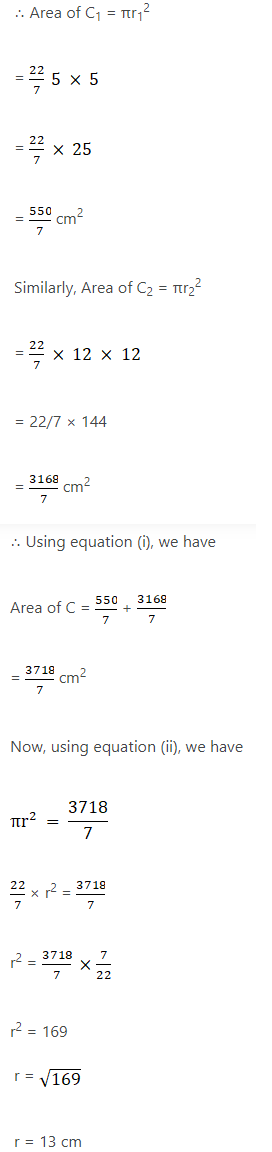⇒ Diameter = 2 × r

= 2 × 13

= 26 cm

Hence, the diameter of the circle is 26 cm.

4. If the area of a circle is numerically equal to twice its circumference, then what is the diameter of the circle?

Solution

Given,

Area of circle = 2 × Circumference of circle …(i)

Let the radius of the circle be ‘r’.

Then, the area of the circle = πr2

and the circumference of the circle = 2πr

Using (i), we have

πr2 = 2 × 2πr

⇒ πr2 = 4 πr

⇒ r = 4 cm

∵ Diameter = 2 × radius

∴ Diameter = 2 × 4

= 8 cm

Hence, the diameter of the circle is 8 cm.

5. What is the perimeter of a square which circumscribes a circle of radius a cm?

Solution

Given,

Perimeter of square circumscribes a circle of radius ‘a’.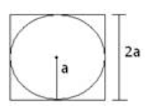Side of square = Diameter of circle

Diameter of circle = 2 × radius

= 2a

So, side of square = 2a

∵ Perimeter of square = 4 × side

∴ Perimeter of square = 4 × 2a

= 8a

Hence, the perimeter of the square is 8a.

6. Find the length of the arc of a circle of diameter 42 cm which subtends an angle of 60° at the centre.

Solution

Given,

Diameter of circle = 42 cm

⇒ Radius of circle = 42/2 cm = 21 cm

Angle subtend at the centre = 60°

∵ Length of arc = θ/360° × 2πr

= 60/360 × 2 × 22/7 × 21

= 22 cm

Hence, the length of the arc is 22 cm.

7. Find the diameter of the circle whose area is equal to the sum of the areas of two circles having radii 4 cm and 3 cm.

Solution

Let the two circles with radii 4 cm and 3 cm be C1 and C2 respectively.

⇒ r1 = 4 cm and r2 = 3 cm

Area of circle, C = Area of C1 + Area of C2 …(i)

∵ Area of circle = πr2 ...(ii)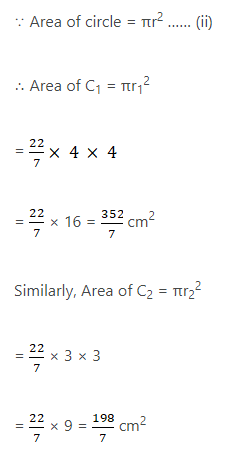So, using (i), we have

Area of C = 352/7 + 198/7 = 550/7 cm2

Now, using (ii), we have

πr2 = 550/7

⇒ 22/7 × r2 = 550/7

⇒ r2 = 550/7 × 7/22 = 25

⇒ r = √25 = 5

⇒ r = 5 cm

∵ Diameter = 2 × radius

∴ Diameter = 2 × 5 = 10 cm

Hence, diameter of the circle with area equal to the sum of two circles of radii 4 cm and 3 cm is 10 cm.

8. Find the area of a circle whose circumference is 8π.

Solution

Given,

Circumference of circle = 8π.

∵ Circumference of a circle = 2πr

∴ 8π = 2πr

⇒ r = 4

∵ Area of circle = πr2

∴ Area of circle = π × 4 × 4

= 16π

Hence, the area of the circle is 16π.

9. Find the perimeter of a semi-circular protractor whose diameter is 14 cm.

Solution

Given,

Diameter of the semi-circular protractor = 14 cm

Radius of the protractor = 14/2 cm = 7 cm

∵ Perimeter of semicircle = πr + d

∴ Perimeter of semicircular protractor = 22/7 × 7 + 14

= 22 + 14

= 36 cm

Hence, the perimeter of the semi-circular protractor is 36 cm.

10. Find the radius of a circle whose perimeter and area are numerically equal.

Solution

Given,

Perimeter of a circle = Area of circle ...(i)

∵ Perimeter of circle = 2πr and Area of circle = πr2

∴ Using (i), we have

2πr = πr2

⇒ 2 = πr2/2πr

⇒ 2 = r or r = 2

Hence, the radius of the circle is 2 cm.

11. The radii of two circles are 19 cm and 9 cm. Find the radius of the circle which has circumference equal to the sum of the circumferences of the two circles.

Solution

Given,

Radius of one of the circles, C1 = 19 cm = r1

Radius of the other circle, C2 = 9 cm = r2

Let the other circle be C with radius ‘r’.

Circumference of C = Circumference of C1 + circumference of C2 …(i)

∵ Circumference of circle = 2πr

∴ Circumference of C1 = 2πr1 = 2 × 22/7 × 19 = 836/7

and circumference of C2 = 2 πr2 = 2 × 22/7 × 9 = 396/7

Using (i), we have

2 πr = 836/7 + 396/7 = 1232/7

⇒ 2 × 22/7 × r = 1232/7

⇒ r = 1232/7 × 7/22 × 1/2 = 28

⇒ r = 28 cm

Hence, the radius of the circle is 28 cm.

12. The radii of two circles are 8 cm and 6 cm. Find the radius of the circle having area equal to the sum of the areas of the two circles.

Solution

Given,

Radius of one of the circles, C1 = 8 cm = r1

Radius of the other circle, C2 = 6 cm = r2

Let the other circle be C with radius ‘r’.

Area of C = Area of C1 + Area of C2 …(i)

∵ Area of circle = πr2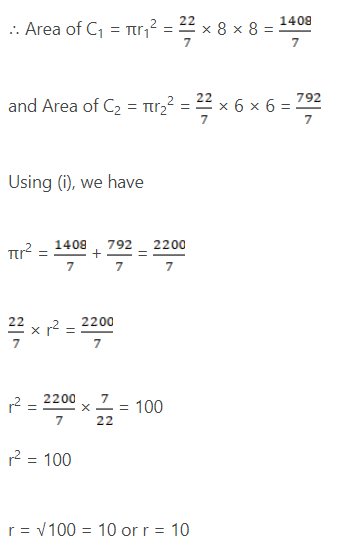Hence, the radius of the circle is 10 cm.

13. Find the area of the sector of a circle having radius 6 cm and of angle 30°.

Solution

Given,

Radius of circle = 6 cm

Angle of the sector = 30°

∵ Area of sector = θ/360 × πr2

= 30/360 × 3.14 × 6 × 6

= 3 × 3.14

= 9.42 cm2

Hence, the area of the sector is 9.42 cm2.

14. In a circle of radius 21 cm, an arc subtends an angle of 60° at the centre. Find the length of the arc.

Solution

Given,

Radius of circle = 21 cm

Angle subtended by the arc = 60°

∵ Length of arc = θ/360 × 2πr

= 60/360 × 2 × 22/7 × 21

= 22 cm

Hence, the length of the arc is 22 cm.

15. The circumference of two circles are in the ratio 2 : 3. What is the ratio between their areas?

Solution

Given,

Ratio of circumferences of two circles = 2 : 3

Let the two circles be C1 and C2 with radii ‘r1’ and 'r2’.

∵ Circumference of circle = 2πr

∴ Circumference of C1 = 2πr1

and circumference of C2 = 2πr2

⇒ 2πr1/2πr2 = 2/3

⇒ r1/r2 = 2/3

Squaring both sides, we get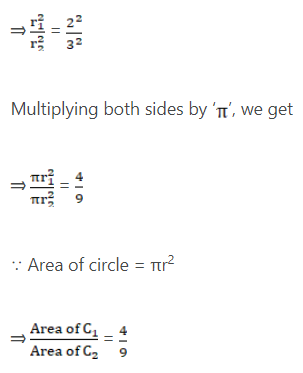Hence, the ratio between the areas of C1 and C2 is 4 : 9.

16. The areas of two circles are in the ratio 4 : 9. What is the ratio between their circumferences ?

Solution

Given,

Ratio of areas of two circles = 2 : 3

Let the two circles be C1 and C2 with radii ‘r1’ and ‘r2’.

∵ Area of circle = πr2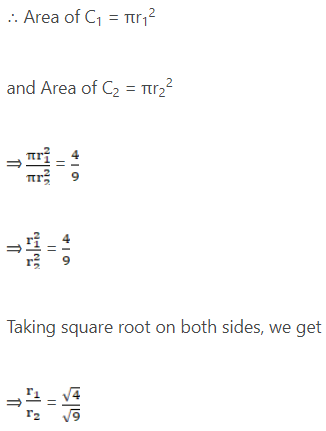⇒ r1/r2 = 2/3

Multiplying and dividing L.H.S. by ‘π’, we get

⇒ πr1/πr2 = 2/3

Multiplying and dividing L.H.S. by ‘2’, we get

⇒ 2πr1/2πr2 = 2/3

As Circumference of circle = 2πr

⇒ Circumference of C1/Circumference of C2 = 2/3

Hence, the ratio between the circumference of C1 and C2 is 2 : 3.

17. A square is inscribed in a circle. Find the ration of the areas of the circle and the square.

Solution

Given,

A square is inscribed in a circle.

Let the radius of circle be ’r’ and the side of the square be ‘x’.

⇒ the length of the diagonal = 2r

∵ Length of side of square = Length of diagonal/√2

∴ Length of side of square = 2r/√2 = √2r

Area of square = side × side = x × r = √2r × √2r = 2r2

Area of circle = πr2

Ratio of areas of circle and square = (Area of circle)/(Area of square) = πr2/2r2 = π/2

Hence, the ratio of areas of circle and square is π : 2.

18. The circumference of a circle is 8 cm. Find the area of the sector whose central angle is 72°.

Solution

Given:

Circumference of circle = 8 cm

Central angle = 72°

∵ Circumference of a circle = 2πr

∴ 2πr = 8

⇒ 2 × 22/7 × r = 8

⇒ r = 8 × 7/22 × 1/2

⇒ r = 14/11 cm

∴ Area of sector = θ/360 × πr2

= 72/360 × π × 14/11 × 14/11

= 1.02 cm2

19. A pendulum swings through an angle of 30° and describes an arc 8.8 cm in length. Find the length of the pendulum.

Solution

Given,

Angle made by the pendulum = 30°

Length of the arc made by the pendulum = 8.8 cm

Then the length of the pendulum is equal to the radius of the sector made by the pendulum.

Let the length of the pendulum be ‘r’.

∵ Length of arc = θ/360 × 2πr

∴ We have,

θ/360 × 2πr = 8.8

⇒ 30/360 × 2 × 3.14 × r = 8.8

⇒ r = 8.8 × 360/30 × 1/2 × 1/3.14

⇒ r = 16.8 cm

Hence, the length of the pendulum is 16.8 cm.

20. The minute hand of a clock is 15 cm long. Calculate the area swept by it in 20 minutes.

Solution

Given,

Length of minute hand = 15 cm

Here, the length of the minute hand is equal to the radius of the sector formed by the minute hand.

Angle made by the minute hand in 1 minute = 360/60 = 6°

Angle made by the minute hand in 20 minutes = 20 × 6 = 120°

Here, the area swept by the minute hand is equal to the area of the corresponding sector made.

∵ Area of sector = θ/360 × πr2

= 120/360 × 3.14 × 15 × 15

= 235.5 cm2

Hence, the area swept by it in 20 minutes is 235.5 cm2.

21. A sector of 56°, cut from a circle, contains 17.6 cm2. Find the radius of the circle.

Solution

Given:

Angle of the sector = 56°

Area of the sector = 17.6 cm2

Let the radius of the circle be ‘r’.

∵ Area of sector = θ/360 × πr2

∴ 17.6 = 56/360 × 22/7 × r2

r2 = 360/56 × 7/22 × 17.6

⇒ r2 = 36

⇒ r = √36

⇒ r = 6 cm

Hence, the radius of the circle is 6 cm.

22. The area of the sector of a circle of radius 10.5 cm is 69.3 cm2. Find the central angle of the sector.

Solution

Given,

Radius of the circle = 10.5 cm

Area of the sector = 69.3 cm2

∵ Area of the sector = θ/360 × πr2

∴ 69.3 = θ/360× 22/7 × 10.5 × 10.5

⇒ θ = 69.3 × 360 × 7/22 × 1/10.5 × 1/10.5

⇒ θ = 72°

Hence, the central angle is 72°.

23. The perimeter of a certain sector of a circle of radius 6.5 cm is 31 cm. Find the area of sector.

Solution

Given,

Radius of circle = 6.5 cm

Perimeter of sector = 31 cm

Now, Perimeter of sector = 2 × radius + Length of arc

∵ Length of arc = θ/360 × 2r × 2πr

∴ Perimeter of sector = 2 × r + θ/360 × 2r × π

= 21 × [1 + θ/360 × π]

31 = 2 × 6.5 × [1 + θ/360 × 22/7]

⇒ 31 = 13 × [1 + θ/360 × 22/7]

⇒ 31/13 = 1 + θ/360 × 22/7

⇒ 31/13 – 1 = θ/360 × 22/7

⇒ 18/13 = θ/360 × 22/7

⇒ θ = 18/13 × 360 × 7/22 …(i)

∵ Area of sector = θ/360 × πr2

∴ Using (i), we have

Area = 18/13 × 360 × 7/22 × 1/360 × 22/7 × 6.5 × 6.5

= 18 × 3.25

= 58.5 cm2

Hence, the area of the sector is 58.5 cm2.

24. The radius of a circle is 17.5 cm. Find the area of the sector enclosed by two radii and an arc 44 cm in length.

Solution

Given,

Radius of circle = 17.5 cm

Length of arc = 44 cm

∵ Length of arc = θ/360 × 2πr

∴ 44 = θ/360 × 2 × 22/7 × 17.5

θ = 44 × 360 × 1/2 × 7/22 × 10/17.5

⇒ θ = 2520/17.5 = 144°

Now, Area of sector = θ/360 × πr2

= 144/360 × 22/7 × 17.5 × 17.5 = 385 cm2

Hence, the area of the sector is 385 cm2.

25. Two circular pieces of equal radii and maximum area, touching each other are cut out from a rectangular cardboard of dimensions 14 cm × 7 cm. Find the area of remaining cardboard.

Solution

Given:

Length of the rectangular cardboard = 14 cm

Breadth of the rectangular cardboard = 7 cm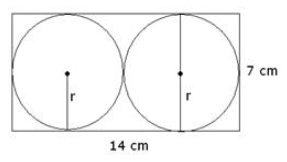∵ Area of rectangle = length × breadth

∴ Area of cardboard = 14 × 7 = 98 cm2

Let the two circles with equal radii and maximum area have a radius of ‘r’ cm. each.

Then, 2r = 7

r = 7/2 cm

∵ Area of circle = πr2

∴ Area of two circular cut outs = 2 × πr2

= 2 × 22/7 × 7/2 × 7/2

= 11 × 7

= 77 cm2

Thus, the area of remaining cardboard = 98 – 77

= 21 cm2

Hence, the area of remaining cardboard is 21 cm2.

26. In the given figure, ABCD is a square of side 4 cm. A quadrant of a circle of radius 1 cm is also drawn. Find the area of the shaded region.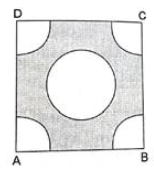Solution

Given,

Side of the square = 4 cm

Radius of the circle in the centre = 1 cm

∵ 4 quadrants = 1 circle

∴ There are 2 circles of radius 1 cm

Area of square = side × side

= 4 × 4

= 16 cm2

Area of 2 circles = 2 × πr2

= 2 × 22/7 × 1 × 1

= 44 cm2

∵ Area of shaded region = Area of square – Area of 2 circles

= 16 - 44/7

= (112 – 44)/7

= 68/7 cm2

= 9.7 cm2

Hence, the area of shaded region is 9.72 cm2.

27. From a rectangular sheet of paper ABCD with AB = 40 cm and AD = 28 cm, semi-circular portion with BC as diameter is cut off. Find the area of the remaining paper.

Solution

Given,

Length of rectangular sheet of paper = 40 cm

Breadth of rectangular sheet of paper = 28 cm

Radius of the semicircular cut out = 14 cm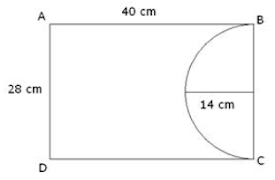∵ Area of rectangle = length × breadth

∴ Area of rectangular sheet of paper = 40 × 28

= 1120 cm2

∵ Area of semicircle = 1/2πr2

∴ Area of semi-circular cut out = 1/2 × 22/7 × 14 × 14

= 22 × 14

= 308 cm2

Thus, the area of remaining sheet of paper = Area of rectangular sheet of paper – Area of semi-circular cut out

= 1120 – 308

= 812 cm2

Hence, the area of remaining sheet of paper is 812 cm2.

28. In the given figure, OABC is a square of side 7 cm. If COPB is a quadrant of a circle with centre C find the area of the shaded region.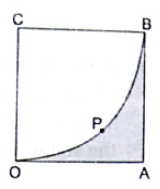Solution

Given:

Side of square = 7 cm

Area of square = side × side

= 7 × 7

= 49 cm2

∵ Area of circle = πr2

∴ Area of a quadrant = 1/4 πr2

= 1/4 × 22/7 × 7 × 7

= 77/2

= 38.5 cm2

Thus, the area of shaded region = Area of square – Area of quadrant

= 49 – 38.5

= 10.5 cm2

Hence, the area of the shaded region is 10.5 cm2.

29. In the given figure, three sectors of a circle of radius 7 cm, making angle of 60°, 80° and 40° at the centre are shaded. Find the area of the shaded region.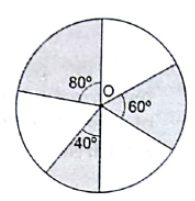Solution

Given,

Radius of circle = 7 cm

Let the sectors with central angles 80°, 60° and 40° be S1, S2, and S3 respectively.

Then, the area of shaded region = Area of S1 + Area of S2 + Area of S3 …(i)

∵ Area of sector = θ/360 × πr2

∴ Area of S1 = 80/360 × 22/7 × 7 × 7

= 308 cm2

Similarly, Area of S2 = 60/360 × 22/7 × 7 × 7

= 154/6 cm2

and area of S3 = 60/360 × 22/7 × 7 × 7

= 154 cm2

Thus, using (i), we have

Area of shaded region = 308/9 + 154/6 + 154/9

= (616 + 462 + 308)/2

= 1386/18

= 77 cm2

Hence, the area of shaded region is 77 cm2.

30. In the given figure, PQ and AB are respectively the arcs of two concentric circles of radii 7 cm and 3.5 cm with centre O. If POQ = 30°, find the area of the shaded region.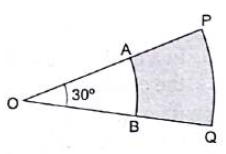Solution

Given,

Radius of inner circle = 3.5 cm

Radius of outer circle = 7 cm

∠POQ = 30°

Let the sector made by the arcs PQ and AB be S1 and S2 respectively.

Then, Area of shaded region = Area of S1 – Area of S2 …(i)

∵ Area of sector = θ/360 × πr2

∴ Area of S1 = 30/360 × 22/7 × 7 × 7

= 71/6 cm2

Similarly, Area of S2 = 30/360 × 22/7 × 3.5 × 3.5

= 77/24 cm2

Thus, using (i), we have

Area of shaded region = 77/6 – 77/24

= (308 – 77)/24

= 231/24

= 77/8 cm2

Hence, the area of shaded region is 77/8 cm2.

31. In the given figure, find the area of the shaded region, if ABCD is a square of side 14 cm and APD and BPC are semicircle.Solution

Given

Side of square = 14 cm

Diameter of each semicircle = 14 cm

Radius of each semicircle = 14/2 = 7 cm

∵ Both are semicircles have same radius.

∴ We consider one circle of radius 7 cm.

Area of shaded region = Area of square – Area of circle …(i)

Area of square = side × side

= 14 × 14

= 196 cm2

Area of circle = πr2

= 22/7 × 7 × 7

= 22 × 7

= 154 cm2.

Thus, using (i), we have

Are of shaded region = 196 – 154

= 42 cm2

Hence, the area of shaded region is 42 cm2.

32. In the given figure, the shape of the top of a table is that of a sector of circle with centre O and AOB = 90°. IF AO = OB = 42 cm, then find the perimeter of the top of the table.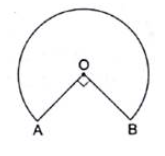Solution

Given:

Radius of the circle = 42 cm

Central angle of the sector = ∠AOB = 90°

Perimeter of the top of the table = Length of the major arc AB + 2 × radius ...(i)

Length of major arc AB = (360 – θ)/360 × 2πr

= (360 – 90)/360 × 2 × 22/7 × 42

= 270/360 × 2 × 22 × 6

= 3/4 × 264

= 3 × 66

= 198 cm

Thus, using (i), we have

Perimeter of the top of the table = 198 + 2 × 42

= 198 + 84

= 282 cm

Hence, the perimeter of the top of the table is 282 cm.

33. In the given figure, ABCD is a square of side 7 cm, DPBA and DQBC are quadrants of circles each of the radius 7 cm. Find the area of shaded region.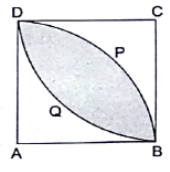Solution

Given:

Side of square = 7 cm

Area of square = side × side

= 7 × 7

= 49 cm2

∵ Area of quadrant = 1/4πr2

∴ Area of 2 quadrants = 2 × 1/4 × πr2

= 1/2 × 22/7 × 7 × 7

= 77 cm2

Area of shaded region = Area of 2 quadrants – Area of square

= 77 – 49

= 28 cm2

Hence, the area of shaded region is 28 cm2.

34. In the given figure, OABC is a quadrant of a circle with centre O and radius 3.5 cm. If OD = 2 cm, find the area of the shaded region.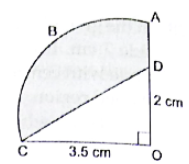Solution

Given,

Radius of Circle = 3.5 cm

OD = 2 cm

∵ Area of Quadrant = 1/4πr2

∴ Area of Quadratic OABC = 1/4 × 22/7 × 3.5 × 3.5

= 9.625 cm2

∵ Area of Triangle = 1/2 × Base × Height

∴ Area of △COD = 1/2 × 3.5 × 2

= 3.5 cm2

Area of Shaded Region = Area of Quadratic OABC – Area of △COD

= 38.5 – 3.5

= 35 cm2

Hence, the area of shaded region is 35 cm2.

35. Find the perimeter of shaded region in the figure, if ABCD is a square of side 14 cm and APB and CPD are semicircles.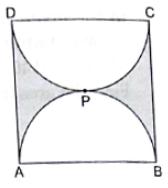Solution

Given,

Side of square = 14 cm

Diameter of semi –circle = 14 cm

⇒ Radius of semi-circle = 14/2 = 7 cm

∵ There are 2 semi circles of same radius.

∴ We consider it as one circle with radius 7 cm.

So,

Perimeter of 2 semicircles = Perimeter of circle = 2πr

= 2 × 22/7 × 7

= 2 × 22

= 44 cm

Perimeter of shaded region = Perimeter of 2 semicircles + 2×Side of square

= 44 + 2 × 14

= 44 + 28

= 72 cm

Hence, the area of the shaded region is 72 cm.

36. In a circle of radius 7 cm, a square ABCD is inscribed. Find the area of the circle which is outside the square.

Solution

Given,

Radius of the circle = 7 cm

Diameter of the circle = 14 cm

Here, diagonal of square = 14 cm

∵ Side of a square = diagonal/√2

⇒ Side = 14/√2

= 7√2 cm

⇒ Area of square = side × side

= 7√2 × 7√2

= 49 × 2

= 98 cm2

Area of circle = πr2

= 22/7 × 7 × 7

= 22 × 7

= 154 cm2
Thus, the area of the circle outside the square = Area of circle – Area of square

= 154 – 98

= 56 cm2

Hence, the area of the required region is 56 cm2.

37. In the given figure, APB and COD are semicircle of diameter 7 cm each, while ARC and BSD are semicircles of diameter 14 cm each. Find the (i) perimeter, (ii) area of the shaded region.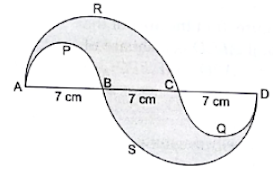Solution

(i) Given,

Diameter of semicircles APB and CQD = 7 cm

⇒ Radius of semicircles ARC and CQD = 7/2 cm = r1

Diameter of semicircles ARC and BSD = 14 cm

Radius of semicircles ARC and BSD = 14/2 cm = 7 cm = r2

Perimeter of APB = Perimeter of CQD

Area of APB = Area of CQD …(i)

Perimeter of ARC = Perimeter of BSD

Area of ARC = Area of BSD …(ii)

∵ Perimeter of semicircle = πr …(iii)

∴ Perimeter of APB = πr1

= 22/7 × 7/2

= 11 cm

Then, using (i), we have

Perimeter of CQD = 11 cm

Now, using (iii), we have

Perimeter of ARC = πr2

= 22/7 × 7

= 22 cm

Then, using (ii), we have

Perimeter of BSD = 22 cm

Perimeter of shaded region = (Perimeter of ARC + Perimeter of APB) + (Perimeter of BSD + Perimeter of CQD)

= (22 + 11) + (22 + 11)

= 33 + 33

= 66 cm

Hence, the perimeter of the shaded region is 66 cm.

(ii) Now,

∵ Area of semicircle = 1/2πr2 …(iv)

= 1/2 × 22/7 × 7 × 7

= 11 × 7

= 77 cm2

Then, by using (ii), we have

Area of BSD = 77 cm2

Area of shaded region = (Area of ARC – Area of APB) + (Area f BSD - Area of CQD)

= (77 – 77/4) + (77 – 77/4)

= (308 – 77)/4 + (308 – 77)/4

= 231/4 + 231/4

= 462/4

= 115.5 cm2

Hence, the area of the shaded region is 115.5 cm2.

38. In the given figure, PSR, RTQ and PAQ are three semicircles of diameter 10 cm, 3 cm and 7 cm respectively. Find the perimeter of shaded region.

Solution

Given,

Diameter of semicircle PSR = 10 cm

⇒ Radius of semicircle PSR = 10/2 = 5 cm = r1

Diameter of semicircle RTQ = 3 cm

⇒ Radius of semicircle RTQ = 3/2 = 1.5 cm = r2

Diameter of semicircle PAQ = 7 cm

⇒ Radius of semicircle PAQ = 7/2 = 3.5 cm = r3

∵ Perimeter of semicircle = πr

∴ Perimeter of semicircle PSR = πr1

= 3.14 × 5

= 15.7 cm

Similarly, Perimeter of semicircle RTQ = πr2

= 3.14 × 1.5

= 4.71 cm

and Perimeter of semicircle PAQ = πr3

= 3.14 × 3.5

= 10.99 cm

Perimeter of shaded region = Perimeter of semicircle PSR + Perimeter of semicircle RTQ + Perimeter of semicircle PAQ

= 15.7 + 4.71 + 10.99

= 31.4 cm

Hence, the perimeter of the shaded region is 3.14 cm.

39. In the given figure, a square OABC is inscribed in a quadrant OPBQ of a circle. IF OA = 20 cm, find the area of the shaded region. [Use π = 3.14]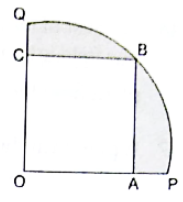Solution

Given,

OA = Side of square OABC = 20 cm

∵ Area of square = Side × Side

∴ Area of square OABC = 20 × 20 = 400 cm2

Now,

∵ Length of diagonal of square = √2 × Side of square

∴ Length of diagonal of square OABC = √2 × 20

= 20√2 cm

∵ Area of quadrant = 1/4 πr2

∴ Area of quadrant OPBQ = 1/4 × 3.14 × 20√2 × 20√2

= 3.14/4 × 400 × 2

= 3.14 × 200

= 628 cm2

Area of shaded region = Area of quadrant OPBQ – Area of square OABC

= 628 – 400

= 228 cm2

Hence, the area of the shaded region is 228 cm2

40. In the given figure, APB and AQO are semicircles and AO = OB. If the perimeter of the figure is 40 cm, find the area of the shaded region.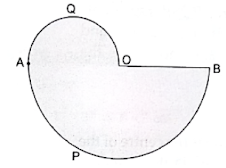Solution

Given,

AO = OB

Perimeter of the figure = 40 cm …(i)

Let the diameters of semicircles AQO and APB be ‘x1’ and ‘x2’ respectively.

Then, using (1), we have

AO = OB

Also, AB = AO + OB

= AO + AO

= 2AO

⇒ x2 = 2x1

So, diameter of APB = 2x1

and diameter of AOQ = x1

and Radius of AOQ = x1/2 …(ii)

Perimeter of shaded region = Perimeter of AQO + perimeter APB + diameter of APB …(iii)

∵ Perimeter of semicircle = πr

∴ Perimeter of semicircle AOQ = 22/7 × x1/2

= 11x1/7 cm

Perimeter of semicircle APB = 22/7 × x1 = 22x1/7 cm

Now, using (iii), we have

40 = 11x1/7 + 22x1/7 + x1

⇒ 40 = (11x1 + 22x1 + 7x1)/7

⇒ 40 × 7 = 40x1

⇒ 280 = 40x1

⇒ x1 = 280/40 = 7 cm

∴ Using (ii), we have

Radius of APB = 7 cm = r1

And Radius of AQO = 7/2 cm

= 3.5 cm

= r2

Now,

∵ Area of semicircle = 1/2πr2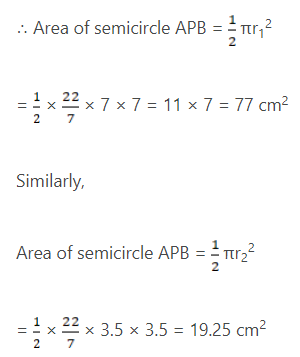Thus, Area of shaded region = Area of APB + Area AQO

= 77 + 19.25

= 96.25 cm2

Hence, the area of the shaded region is 96.25 cm2.

41. Find the area of a quadrant of a circle whose circumference is 44 cm.

Solution

Given,

Circumference of circle = 44 cm

Let the radius of the circle be ’r’ cm

∵ Circumference of circle = 2πr

∴ 44 = 2πr

⇒ 44/2 = 22/7 × r

⇒ r = 22 × 7/22 = 7 cm

Now, Area of quadrant = 1/4 × πr2

= 1/4 × 22/7 × 7 × 7

= (11 × 7)/2

= 22/7

= 38.5 cm2

Hence, the area of the quadrant is 38.5 cm2.

42. In the given figure, find the area of the shaded region, where ABCD is a square of side 14 cm and all circle are of the same diameter.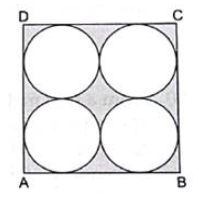Solution

Given:

Side of square = 14 cm

Let the radius of each circle be ‘r’ cm

Then, 2r + 2r = 14 cm

4r = 14 cm

⇒ r = 14/4

⇒ r = 7/2

Area of square = side × side

= 14 × 14

= 196 cm2

∵ Area of circle = πr2

∴ Area of 4 circles = 4 × πr2

= 4 × 22/7 × 7/2 × 7/2

= 22 × 7

= 154 cm2

Area of shaded region = Area of the square – Area of 4 circles

= 196 – 154

= 42 cm2

Hence, the area of the shaded region is 42 cm2.

43. Find the area of the shaded region in the given figure, if ABCD is a rectangle with sides 8 cm and 6 cm and O is the centre of the circle.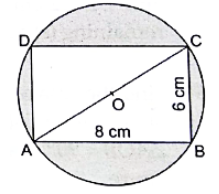Solution

Given,

Length of rectangle = 8 cm

Breadth of rectangle = 6 cm

Area of rectangle = length × breadth

= 8 × 6

= 48 cm2

Consider △ABC,

By Pythagoras theorem,

AC2 = AB2 + BC2

= 82 + 62

= 64 + 36

= 100

AC = √100 = 10 cm

⇒ Diameter of circle = 10 cm

Thus, radius of circle = 10/2 = 5 cm

Let the radius of circle be r = 5 cm

Then, Area of circle = πr2

= 22/7 × 5 × 5

= (22 × 25)/7

= 550/7

= 78.57 cm2

Area of shaded region = Area of circle – Area of rectangle = 78.7 – 48

= 30.57 cm2

Hence, the area of shaded region is 30.57 cm2

44. A wire is bent to from a square enclosing an area of 484 m2. Using the same wire, a circle is formed. Find the area of the circle.

Solution

Given,

Perimeter of square = Circumference of circle …(i)

Area of square = 484 m2

Let the side of square be ‘x’ cm.

∵ Area of Square = side × side

∴ 484 = x × x

⇒ x2 = 484

⇒ x = √484 = 22 cm

∵ Perimeter of square = 4 × side

= 4 × 22

= 88 cm

∴ Using (i), we have

Circumference of circle = 88 cm

Also, Circumference of Circle = 2πr

2πr = 88

⇒ 2 × 22/7 × r = 88

⇒ r = 88 × 1/2 × 7/22

⇒ r = 2 × 7 = 14 cm

Area of Circle = πr2 = 22/7 × 14 × 14

= 22 × 2 × 14

= 616 cm2

Hence, the area of Circle is 616 cm2.

45. A square ABCD is inscribed in a circle of radius ‘r’. Find the area of the square.

Solution

Given,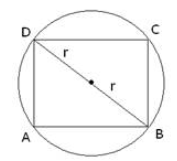Diagonal of Square = 2r

∵ Side of square = (length of diagonal)/√2

∴ Side = 2r/√2 = √2r

Area of square = Side × Side

= √2r × √2r

= 2r2

Hence, the area of square is ‘2r2’ square units.

46. The cost of fencing a circular field at the rate of Rs 25 per meter is Rs 5500. The field is to be ploughed at the rate of 50 paise per m2. Find the cost of ploughing the field. [Take π = 22/7]

Solution

Given,

Rate of fencing a circular field = Rs 25/m

Cost of fencing a circular field = RS 5500

Rate of ploughing the field = 50p/m2 = RS 0.5 m2

Let the radius of circular field be ‘r’ and the length of the field fenced be ‘x’ m.

Then, 25 × x = 5500

x = 5500/225

= 220 m

∵ Circumference of circular field = 2 πr

∴ 220 = 2πr

220 = 2 × 22/7 × r

⇒ r = (220 × 7)/(2 × 22)

⇒ r = 35 m

Area of the circular field = πr2

= 22/7 × 35 × 35

= 22 × 5 × 35

= 3850 m2

Now, cost of ploughing the field = Rate of ploughing the field × Area of the field

= 0.5 × 3850

= Rs 1925

Hence, the cost of Ploughing the field is Rs 1925.

47. A park is in the form of a rectangle 120 m by 90 m. At the centre of the park, there is a circular lawn as shown in the figure. The area of the park excluding the lawn is 2950 m2. Find the radius of the circular lawn. [Given, π = 3.14]

Solution

Given,

Length of the rectangular park = 120 m

Breadth of the rectangular park = 90 m

Area of the park excluding the circular lawn = 2950 m2

Area of rectangular park = length × breadth

= 120 × 90

= 10800 m2

Area of circular lawn = Area of rectangular park – Area of park excluding the lawn

= 10800 – 2950

= 7850 m2

∵ Area of circle = πr2

∴ 7850 = 3.14 × r2

r2 = 7850/3.14 = 2500

⇒ r = 2500 = 50 m

Hence, the radius of the circular lawn is 50 m.

48. In the given figure PQRS represents a flower be. If OP = 21 m and OR = 14 m, find the area of the flower bed.

Solution

Given,

OP = 21 m = r1

OR = 14 m = r2

Let the quadrants made by outer and inner circles be Q1 and Q2, with radius r1 and r2 respectively.

Then, Area of flower bed = Area of Q1 – Area of Q2

∵ Area of Quadrant = 1/4πr2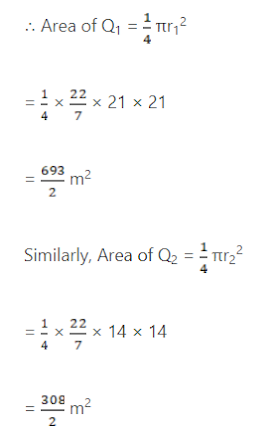Thus, Area of flower bed = 693/2 – 308/2

= 385/2

= 192.5 m2

Hence, the area of the flower bed is 192.5 m2.

49. In the given figure, O is the centre of the bigger circle, and AC is its diameter. Another circle with AB as diameter is drawn. If AC = 54 cm and BC = 10 cm, find the area of the shaded region.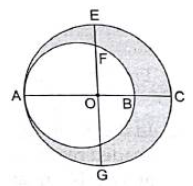Solution

Given,

AC = 54 cm

BC = 10 cm

⇒ AB = AC – BC = 54 – 10

= 44 cm

Radius of bigger circle = AC/2 = 54/2 = 27 cm = r1

Radius of Smaller circle = AB/2 = 44/2 = 22 cm = r2

∵ Area of circle = πr2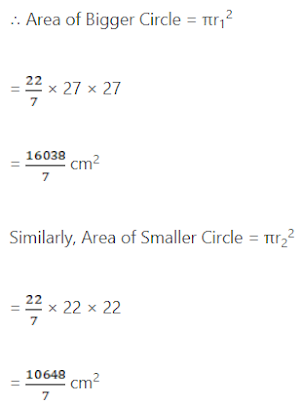Area of shaded region = Area of Bigger Circle – Area of smaller circle = 16038/7 – 10648/7

= 5390/7

= 770 cm2

Hence, area of shaded region is 770 cm2.

50. From a thin metallic piece in the shape of a trapezium ABCD in which AB|| CD and BCD = 90°, a quarter circle BFEC is removed. Given, AB = BC = 3.5 cm and DE = 2 cm, calculate the area of remaining (shaded) part of metal sheet.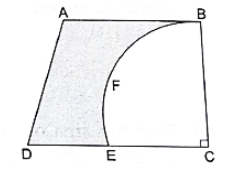Solution

Given,

AB || CD

∠BCD = 90°,

AB = BC = 3.5 cm = EC

DE = 2 cm

DC = DE + EC = 2 + 3.5 = 5.5 cm

Area of Trapezium = 1/2 × Sum of Parallel Sides × h

= 1/2 × (AB + DC) × BC

= 1/2 × (3.5 + 5.5) × 3.5

= 1/2 × 9 × 3.5

= 15.75 cm2

Area of quadrant BEFC = 1/4 × πr2

= 1/4 × 22/7 × 3.5 × 3.5

= 9.625 cm2

Thus, Area of remaining part of metal sheet = Area of Trapezium – Area of Quadrant BEFC

= 15.75 – 9.625

= 6.125 cm2

Hence, the area of the remaining part of metal sheet is 6.125.

51. Find the area of the major segment APB of a circle of radius 35 cm and AOB = 90°, as shown in the given figure.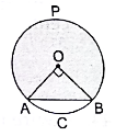Solution

Given,

Radius of Circle = 35 cm

∠AOB = 90°

∵ Area of Sector = θ/360 × πr2

= 90/360 × 22/7 × 35 × 35

= 1925/2 cm2

∵ △AOB is right-angled triangle.

∴ Area of △AOB = 1/2 × OA × OB

= 1/2 × 35 × 35

= 1225/2 cm2

Now, Area of Minor Segment ACB = Area of sector – Area of △AOB

= 1925/2 – 1225/2

= 700/2

= 350 cm2

Area of circle = πr2

= 22/7 × 35 × 35

= 22 × 5 × 35

= 3850 cm2

Thus, Area of Major Segment = Area of Circle – Area of Minor segment

= 3850 – 350

= 3500 cm2

Hence, the area of the major segment is 3500 cm2.